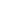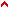1.3 Part 3 - multiplying figures
This part is about figures derived from the prime ones, here we show how some figures are derived from others, and how one figure can be measured with another; and we show how some parts are equivalent to others in one and the same figure.Top